# Universal Logic Gate NOR

Logic Gate

In this tutorial we will learn about the Universal Logic Gate NOR.

## Universal Gate

A universal gate is a logic gate which can be used to implement any other type of logic gates.

## Logic Gate: NOR

The logic gate NOR takes two or more input and works as per the following truth table.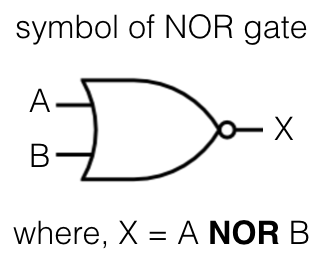## NOR gate truth table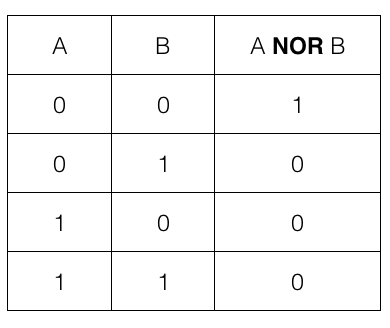i.e., if both the input A and B are 0 or FALSE then the output is 1 or TRUE otherwise it is 0 or FALSE.

We can create AND, OR and NOT gate using the NOR gate.

## NOT gate using NOR gate

We need only one NOR gate to implement a NOT gate. Let us first check a NOT gate and its truth table.

The logic gate NOT takes only one input and works as per the following truth table.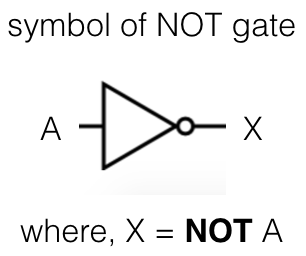## NOT gate truth table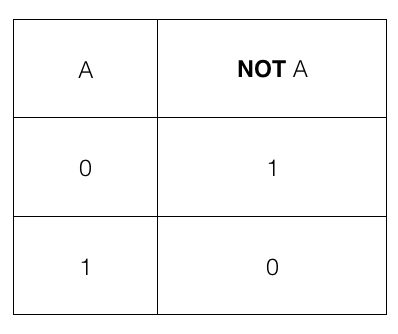i.e., if the input A is 1 or TRUE then the output is 0 or FALSE and if the input A is 0 or FALSE then the output is 1 or TRUE

## NOR gate to create a NOT gate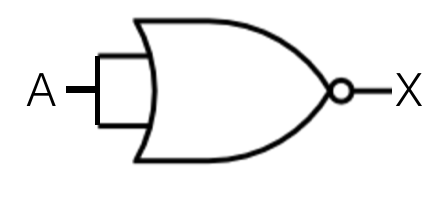where, X = A NOR A

So, we feed A as input to the NOR gate and the output X is the final result which is same as a NOT gate.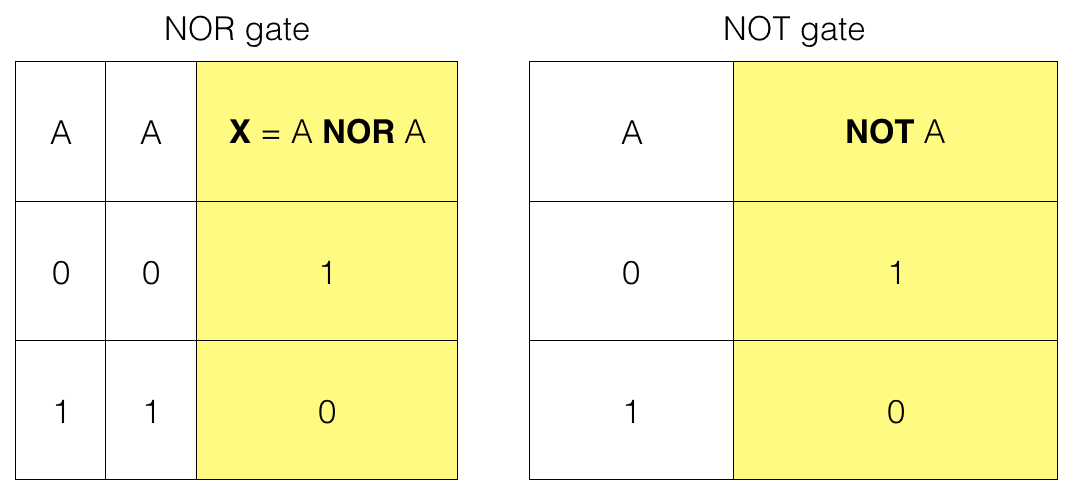So, we have a NOT gate using a NOR gate

## OR gate using NOR gate

We need two NOR gates to implement an OR gate. Let us first check an OR gate and its truth table.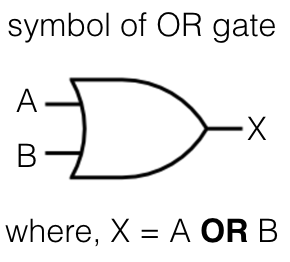## OR gate truth table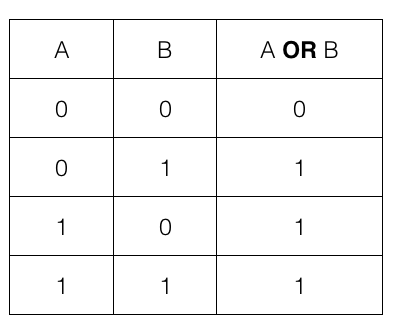i.e., if any one of the input A or B is 1 or TRUE then the output is 1 or TRUE; otherwise it is 0 or FALSE.

## NOR gates to create an OR gate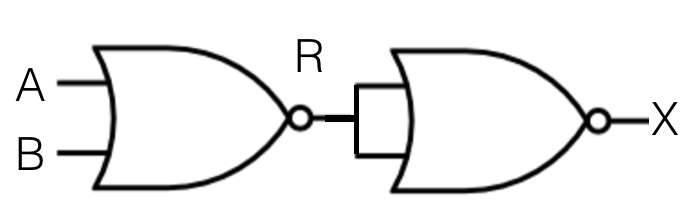where, R = A NOR B

and, X = R NOR R

So, we feed A and B as input to the first NOR gate. The output of the first NOR gate R then becomes input for the second NOR gate and the output of the second NOR gate X is the final result which is same as an OR gate.Note! For the second NOR gate both the input is equal to R.

So, we have an OR gate result using two NOR gates.

## AND gate using NOR gate

We need three NOR gates to implement an AND gate. Let us first check an AND gate and its truth table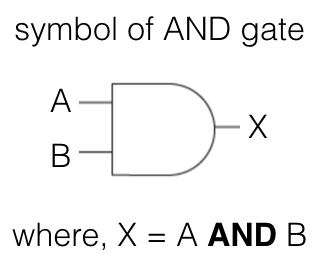## AND gate truth table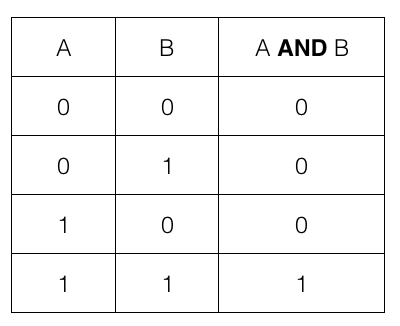i.e., if both the input A and B are 1 or TRUE then the output is 1 or TRUE; otherwise it is 0 or FALSE

## NOR gates to create an AND gate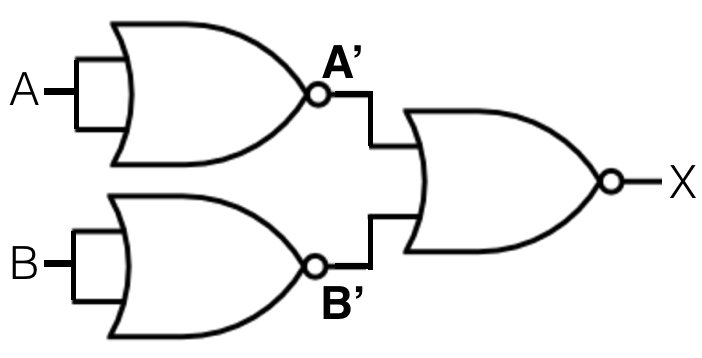where, A' = A NOR A

and, B' = B NOR B

and, X = A' NOR B'

So, we feed A as input to the first NOR gate and B as input to the second NOR gate. The output of the first NOR gate A' and the output of the second NOR gate B' becomes input for the third NOR gate and output of the third NOR gate X is equivalent to an AND gate.

Truth table of the First NOR gate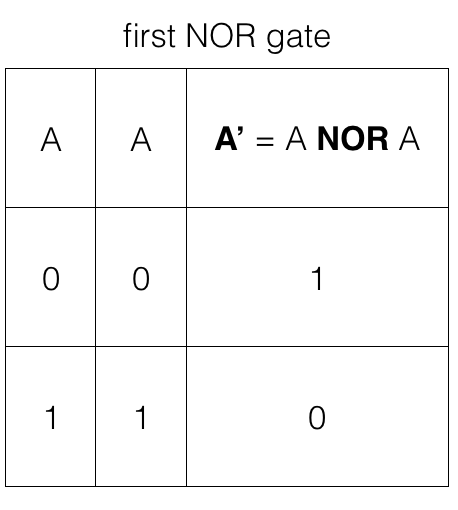Truth table of the second NOR gate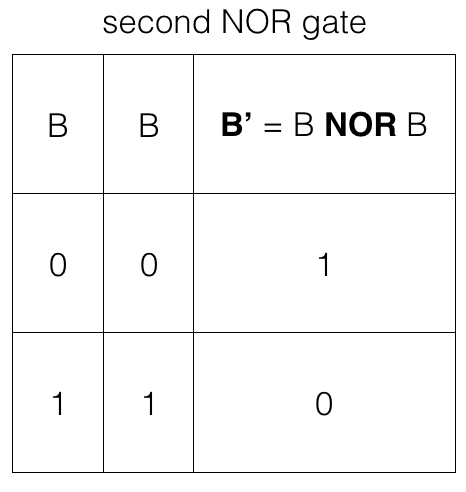Note! The third NOR gate takes A' and B' as input so, we have four input options.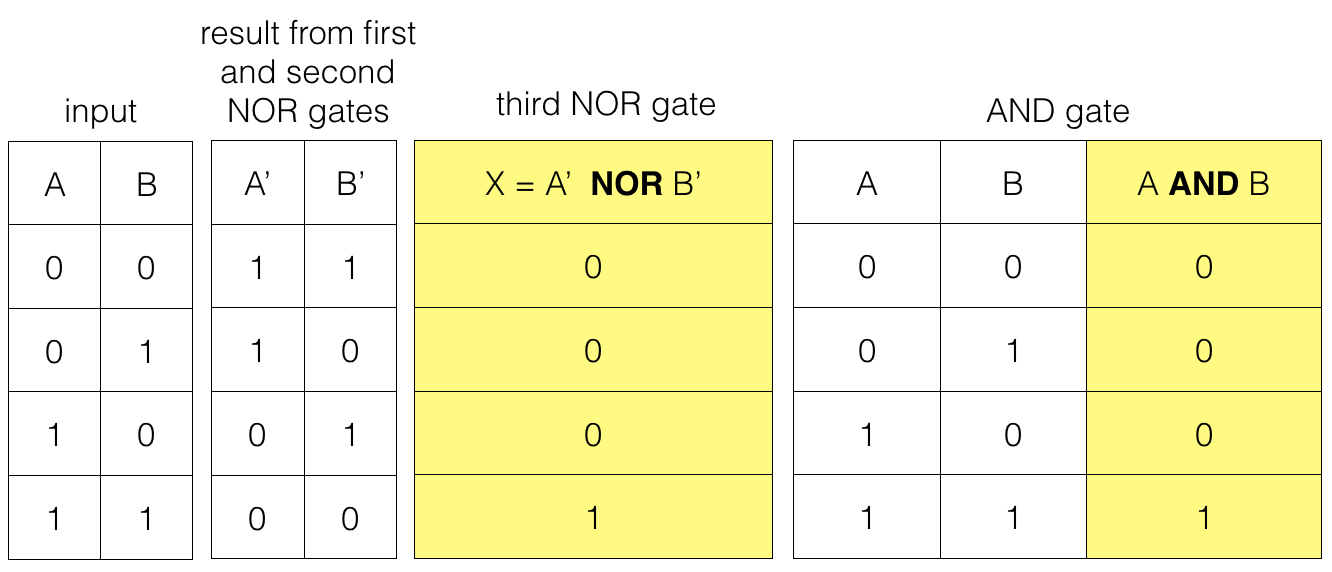So, we have the result of an AND gate using three NOR gates.